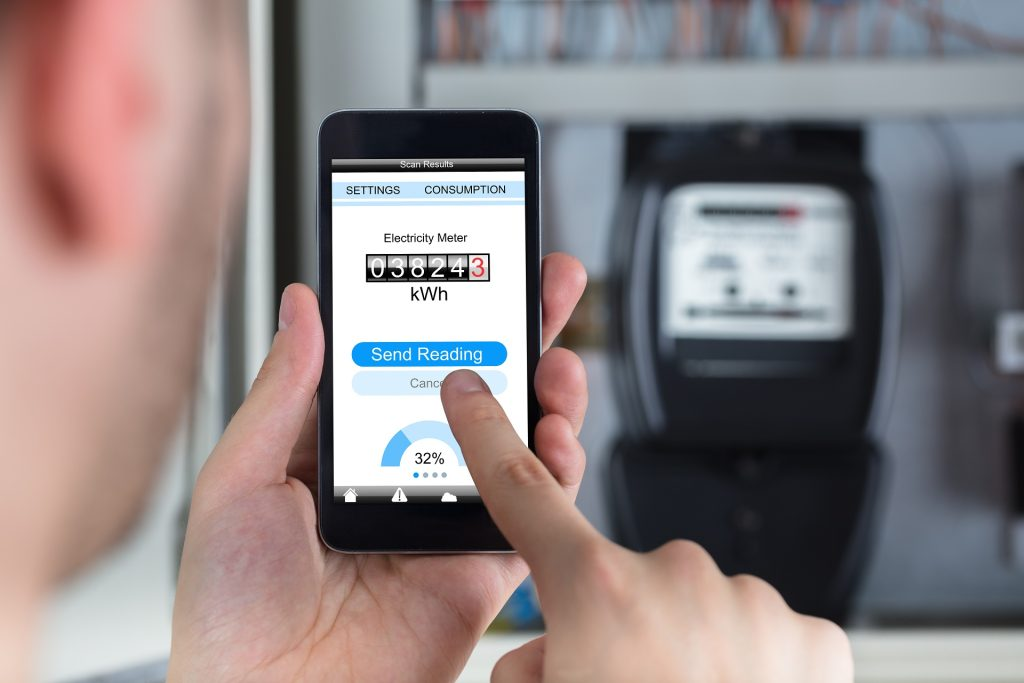# Do Heated Floors Use A Lot of Electricity?Radiant heat does not use a lot of electricity. This post goes into more detail about why that is and some methodology you can use to project costs. Portland-area homeowners will find this post especially useful.

One of the most common concerns about radiant heat flooring is the amount of energy the system will consume and its comparison to other services. The good news is, not only is radiant heat comparable, but the efficiency of the system will likely save you money in the long run. Below, we get into some of the specifics about the amount of electricity heated floors use.

### Heated Floor Electricity Specifics

Most heated tile floors and electric floor heating systems use 12 watts per hour per square foot, meaning a 100-square-foot room would use 1200 watts in total every hour (potentially up to 300 watts less than the average space heater). This means the even, efficient heat of a floor heating system would potentially cost less almost immediately.

### How To Calculate Radiant Heat Costs

By following this process, you can get a potential estimate of costs:

1. Calculate the square footage of the heated area in your space. You can get an estimate of this by multiplying the square footage of the whole room by 0.9.
2. Multiply the heated area by 12 since most systems use 12 watts per square foot.
3. Now divide the total number of watts by 1000 to get the number of kilowatts the system will use per hour. (KW is the unit of measurement that electric companies use when determining monthly bills.)
4. Finally, multiply the number of kilowatts used per hour by how much the electric company charges per kilowatt in your area. Average costs are around .12 per KW. (Keep in mind this pricing may fluctuate slightly in peak hours or in extreme heat cases.)